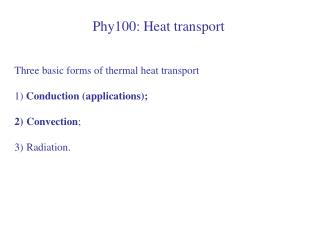DownloadDownload PresentationPhy100: Heat transport

# Phy100: Heat transport

Download Presentation## Phy100: Heat transport

- - - - - - - - - - - - - - - - - - - - - - - - - - - E N D - - - - - - - - - - - - - - - - - - - - - - - - - - -
##### Presentation Transcript

1. Phy100: Heat transport • Three basic forms of thermal heat transport • 1) Conduction (applications); • Convection; • Radiation.

2. Heat conduction Consider an old brick house. The brick thickness. Outside winter temperature T=0. Inside temperature is kept at 24c. 1) Assume the house is heated by Natural gases. Estimate the amount of natural gases used each month in units of GJ ? 2) How much energy can one save by lowering the inside temperatures ?

3. Energy model for house heating(dynamical equilibrium) Energy flows Out by heat conduction, and radiation House At a constant temperature or With constant thermal energy Energy flows in by burning natural gases, or consuming electricity E (in) = E(out) Or Power (in) = Power (out)

4. Approach • Calculate the outgoing energy (conduction loss rate) (you need to know the surface area, thickness and materials etc). 2) To maintain a constant temperature in a house, the conduction loss has to be balanced by the energy supplied by natural gases. 3) This can be converted into the amount of natural gases needed per day or per month.

5. Q1: Estimate the conducted energy Kappa for red brick is 0.6 W/m. K. • Area is 200m^2, T inside=24C, T outside = 0C, thickness=30cm, • The conduction power is • 480 W; • B) 3000W; • C) 5000W; • D) 9600W.

6. Solution A=200m^2, T inside – T outside = 24K, d=0.3m, Q/t = 9600 W. Q for one day is 829,440 KJ. Q for one month is 24,883,200 KJ = 24.9 GJ Terasen Gas uses GJ (=billion J) as units. 1GJ charge is about 10 CAD. Monthly bill for house heating is about 250CAD.